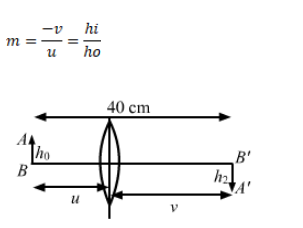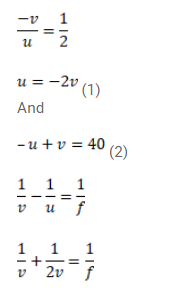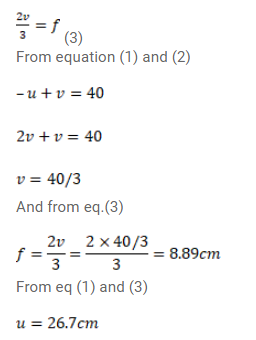# A pin of length 2cm is placed perpendicular to the principal axisQuestion:

A pin of length $2 \mathrm{~cm}$ is placed perpendicular to the principal axis of a converging lens. An inverted image of size $1 \mathrm{~cm}$ is formed at a distance of $40 \mathrm{~cm}$ from the pin. Find the focal length of the lens and its distance from the pin.

Solution: